* Log In to use the Calculate function * Become a Member!

###### Civil and Structural Engineering Design Calculations: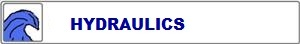• Hydrology calculations, open channel calculations, culvert calculations, sewer calculations and water force main calculations.• Traffic and road geometry calculations, surveying and earthwork calculations, pavement calculations and concrete floors-on-grade calculations.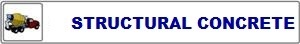• Shallow foundation calculations, concrete cantilever retaining wall calculations, concrete column calculations, concrete beam calculations and suspended concrete floor calculations.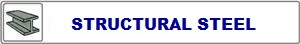• Steel shape properties, steel column calculations, steel beam calculations, steel tension member calculations, steel connector calculations and steel welding calculations.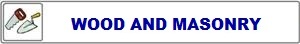• Load Resistance Factor Design, LRFD, Structural wood calculations, Allowable Stress Design, ASD, Structural wood calculations and ASD concrete masonry calculations.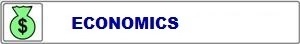• Engineering Economics Calculations. Perform calculations for engineering economics such as present worth, compound amount, uniform gradiant, sinking fund and capital recovery.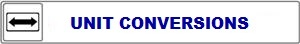• Engineering unit conversions. Convert units of measurement, English or Metric.

 Start Using The Calculations >>> Become A Member Now!